# CHANGING THE STATE OF A SYSTEM WITH HEAT AND WORK

- Changes in the state of a system are produced by interactions with the environment through heat and work.

- During these interactions, equilibrium (a static or quasi-static process) is necessary for the equations that relate system properties to one-another to be valid.

- Heat and work are transfers of energy across a system boundary

1. ## Changing the State of a System : Heat(VW, S & B: 4.7-4.9)

1. ### Heat is energy transferred between a system and its surroundings by virtue of a temperature difference only.

1. This transfer of energy can change the state of the system.
2. "Adiabatic" means no heat is transferred.

### Zeroth Law of Thermodynamics (VW, S & B: 2.9-2.10)

1. There exists for every thermodynamic system in equilibrium a property called temperature. (Absolute temperature scales: K = 273.15+oC, R = 459.9 +oF)
2. Equality of temperature is a necessary and sufficient condition for thermal equilibrium, i.e. no transfer of heat.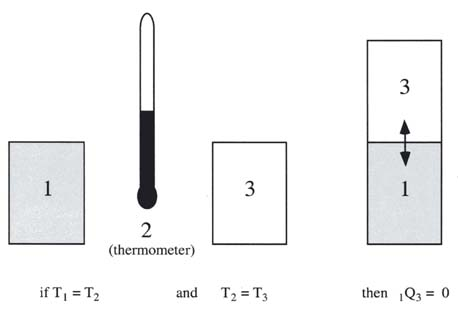2. ## Changing the State of a System: Work(VW, S & B: 4.1-4.6)

1. ### Definition of Work

1. We saw that heat is a way of changing the energy of a system by virtue of a temperature difference only.
2. Any other means for changing the energy of a system is called work. We can have push-pull work (e.g. in a piston-cylinder, lifting a weight), electric and magnetic work (e.g. an electric motor), chemical work, surface tension work, elastic work, etc.
3. In defining work, we focus on the effects that the system (e.g. an engine) has on its surroundings. Thus we define work as being positive when the system does work on the surroundings (energy leaves the system). If work is done on the system (energy added to the system), the work is negative.

### Consider a simple compressible substance

Work done by system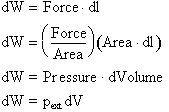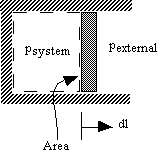therefore: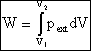or in terms of the specific volume, v: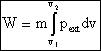where m is the mass of the system.1. If system volume expands against a force, work is done by the system.

2. If system volume contracts under a force, work is done on the system.

3. Why pexternal instead of psystem?

Consider pexternal = 0 (vacuum). No work is done by the system even though psystem changes and the system volume changes.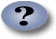### Quasi-static processes

Use of pext instead of psys is often inconvenient because it is usually the state of the system that we are interested in.

However, for quasi-static processes psys Å pext..

Consider pext = psys ± dp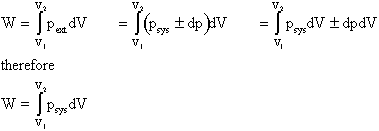is the work done by the system in a quasi-static process.

1. Can only relate work to system pressure for quasi-static processes.

2. Take a free expansion (pext = 0) for example: psys is not related to pext ( and thus the work) at all -- the system is not in equilibrium.

2. Work is a path dependent process
1. Work depends on path

2. Work is not a function of the state of a system

3. Must specify path if we need to determine work

4.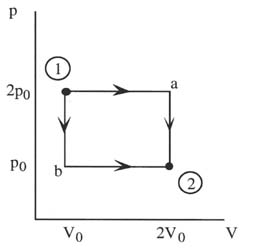Along Path a: W = 2p0(2V0 - V0) = 2p0V0

Along Path b: W = p0(2V0 - V0) = p0V0

5. Example: Work during quasi-static, isothermal expansion of a thermally perfect gas from p1, V1 to p2, V2 .

First, is path specified? Yes -- isothermal.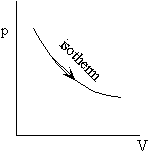Equation of state for thermally perfect gas

pV = nRT n = number of moles

R = Universal gas constant

V = total system volume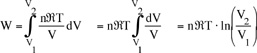also for T = constant, p1V1 = p2V2 , so the work done by the system is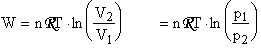or in terms of the specific volume and the system mass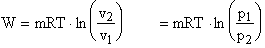3. Work vs. heat transfer -- which is which?
1. Can have one, the other, or both. It depends on what crosses the system boundary. For example consider a resistor that is heating a volume of water.

2.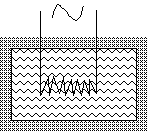3. If the water is the system, then the state of the system will be changed by heat transferred from the resistor.

4. If the system is the water + the resistor, then the state of the system will be changed by (electrical) work.